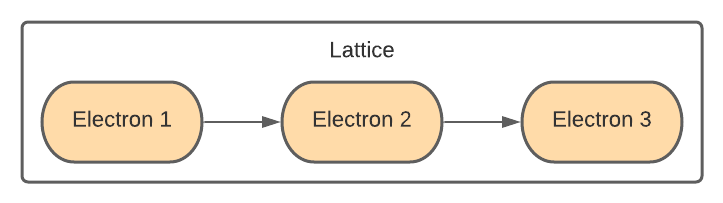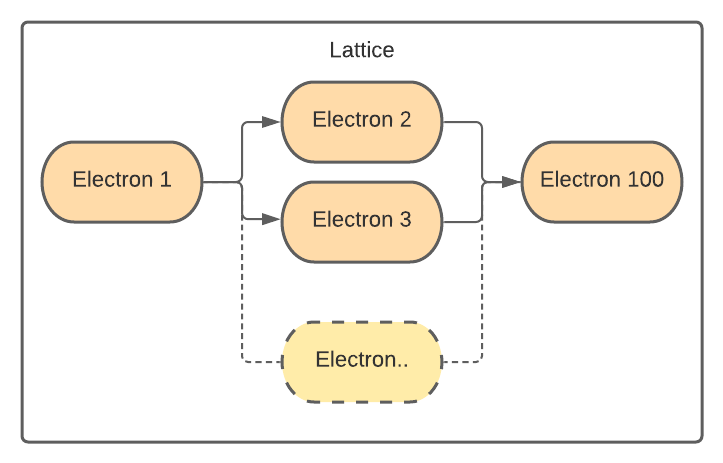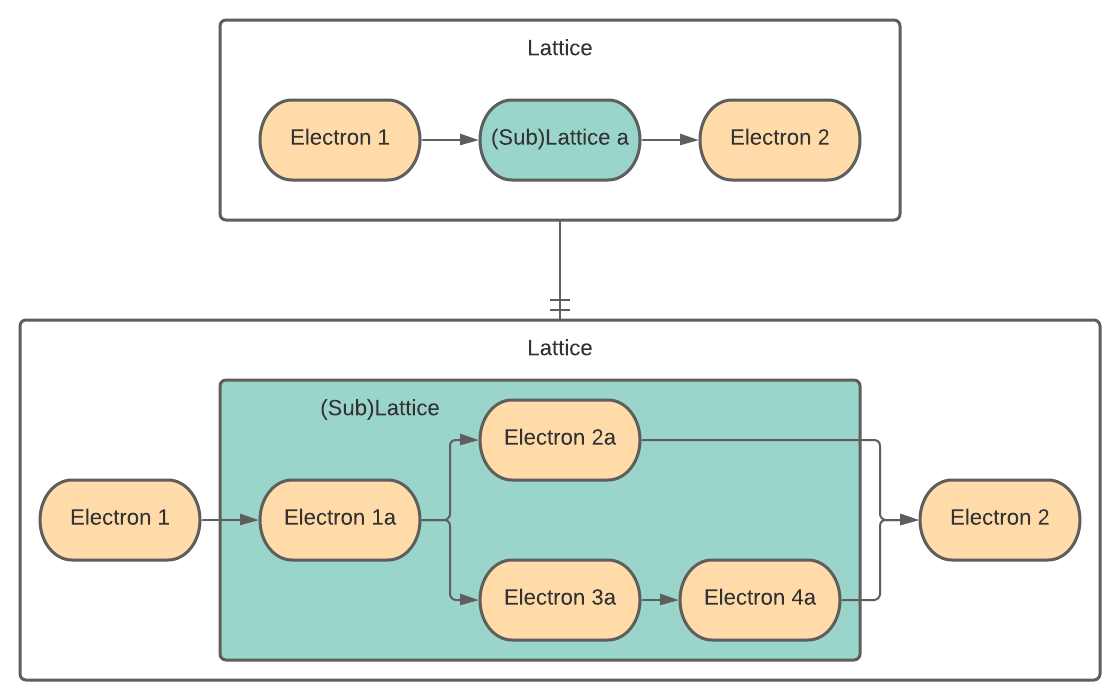# The Covalent SDK#

The Covalent SDK exists to enable compute-intensive workloads, such as ML training and testing, to run as server-managed workflows. To accomplish this, the workload is broken down into tasks that are arranged in a workflow. The tasks and the workflow are Python functions decorated with Covalent’s electron and lattice interfaces, respectively.

## Electron#

The simplest unit of computational work in Covalent is a task, called an electron, created in the Covalent API by using the `@covalent.electron` decorator on a function.

In discussing object-oriented code, it’s important to distinguish between classes and objects. Here are notational conventions used in this documentation:

`Electron` (capital “E”)

The Covalent API class representing a computational task that can be run by a Covalent executor.

electron (lower-case “e”)

An object that is an instantiation of the `Electron` class.

`@covalent.electron`

The decorator used to (1) turn a function into an electron; (2) wrap a function in an electron, and (3) instantiate an instance of `Electron` containing the decorated function (all three descriptions are equivalent).

The `@covalent.electron` decorator makes the function runnable in a Covalent executor. It does not change the function in any other way.

The function decorated with `@covalent.electron` can be any Python function; however, it should be thought of, and operate as, a single task. Best practice is to write an electron with a single, well defined purpose; for example, performing a single tranformation of some input or writing or reading a record to a file or database.

Here is a simple electron that adds two numbers:

```import covalent as ct

@ct.electron
return x + y
```

An electron is a building block, from which you compose a lattice.## Lattice#

A runnable workflow in Covalent is called a lattice, created with the `@covalent.lattice` decorator. Similarly to electrons, here are the notational conventions:

`Lattice` (capital “L”)

The Covalent API class representing a workflow that can be run by a Covalent dispatcher.

`lattice` (lower-case “l”)

An object that is an instantiation of the `Lattice` class.

`@covalent.lattice`

The decorator used to create a lattice by wrapping a function in the `Lattice` class. (The three synonymous descriptions given for electron hold here as well.)

The function decorated with `@covalent.lattice` must contain one or more electrons. The lattice is a workflow, a sequence of operations on one or more datasets instantiated in Python code.

For Covalent to work properly, the lattice must operate on data only by calling electrons. By “work properly,” we mean “dispatch all tasks to executors.” The flexibility and power of Covalent comes from the ability to assign and reassign tasks (electrons) to executors, which has two main advantages, hardware independence and parallelization.

Hardware indepdendence

The task’s code is decoupled from the details of the hardware it is run on.

Parallelization

Independent tasks can be run in parallel on the same or different backends. Here, indepedent means that for any two tasks, their inputs are unaffected by each others’ execution outcomes (that is, their outputs or side effects). The Covalent dispatcher can run independent electrons in parallel. For example, in the workflow structure shown below, electron 2 and electron 3 are executed in parallel.Note

A function decorated as an electron behaves as a regular function unless called from within a lattice. Only when an electron is invoked from within a lattice is the Electron code invoked to run the function in an executor.

Also note

When an electron is called from another electron, it is executed as a normal Python function. That is, the calling electron (if run in a lattice) is assigned to an executor, but the inner electron runs as part of the calling electron – it is not farmed out to its own executor.

The example below illustrates this simple but powerful paradigm. The tasks are constructed first using the `@covalent.electron` decorator, then the `@covalent.lattice` decorator is applied on the workflow function that manages the tasks.

```# ML example: electrons and lattice

from numpy.random import permutation
from sklearn import svm, datasets
import covalent as ct

@ct.electron
perm = permutation(iris.target.size)
iris.data = iris.data[perm]
iris.target = iris.target[perm]
return iris.data, iris.target

@ct.electron
def train_svm(data, C, gamma):
X, y = data
clf = svm.SVC(C=C, gamma=gamma)
clf.fit(X[90:], y[90:])
return clf

@ct.electron
def score_svm(data, clf):
X_test, y_test = data
return clf.score(X_test[:90], y_test[:90])

@ct.lattice
def run_experiment(C=1.0, gamma=0.7):
clf = train_svm(data=data, C=C, gamma=gamma)
score = score_svm(data=data, clf=clf)
return score
```

Notice that all the data manipulation in the lattice is done by electrons. The How-to Guide contains articles on containing data manipulation within electrons.

## Sublattice#

It is common practice to perform a nested set of experiments. For example, you design an experiment from a set of tasks defined as electrons. You construct the experiment as a lattice, then dispatch the experiment using some test parameters.

Now assume that you want to run a series of these experiments in parallel across a spectrum of input parameters. Covalent enables exactly this technique through the use of sublattices.

A sublattice is a lattice transformed into an electron by applying an electron decorator after applying the lattice decorator.

For example, the lattice `experiment` defined below performs some experiment for a given set of parameters. To carry out a series of experiments for a range of parameters, you wrap the `experiment` lattice with the `@electron` decorator to construct the `run_experiment` sublattice. (The example below explicitly calls `electron` to wrap `experiment` rather than using “@” notation. The result is the same.)

When `run_experiment_suite` is dispatched for execution, it runs the experiment with an array of different input parameter sets. Since this arrangement meets the criteria for independence of the sublattices’ inputs and outputs, Covalent executes the sublattices in parallel!

```@ct.electron
...

@ct.electron
...

@ct.lattice
def experiment(**params):
return final_result

run_experiment = ct.electron(experiment) # Construct a sublattice

@ct.lattice
def run_experiment_suite(**params):
res = []
for param in params:
res.append(run_experiment(**params))
return res
```

Conceptually, as shown in the figure below, executing a sublattice adds the constituent electrons to the transport graph.Note

Don’t confuse `ct.electron(lattice)`, which creates a sublattice, with `ct.lattice(electron)`, which is a workflow consisting of a single task.

## Dispatch#

You dispatch a workflow in your Python code using the Covalent `dispatch()` function. For example, to dispatch the `run_experiment` lattice in the ML example:

```# Send the run_experiment() lattice to the dispatch server
dispatch_id = ct.dispatch(run_experiment)(C=1.0, gamma=0.7)
```